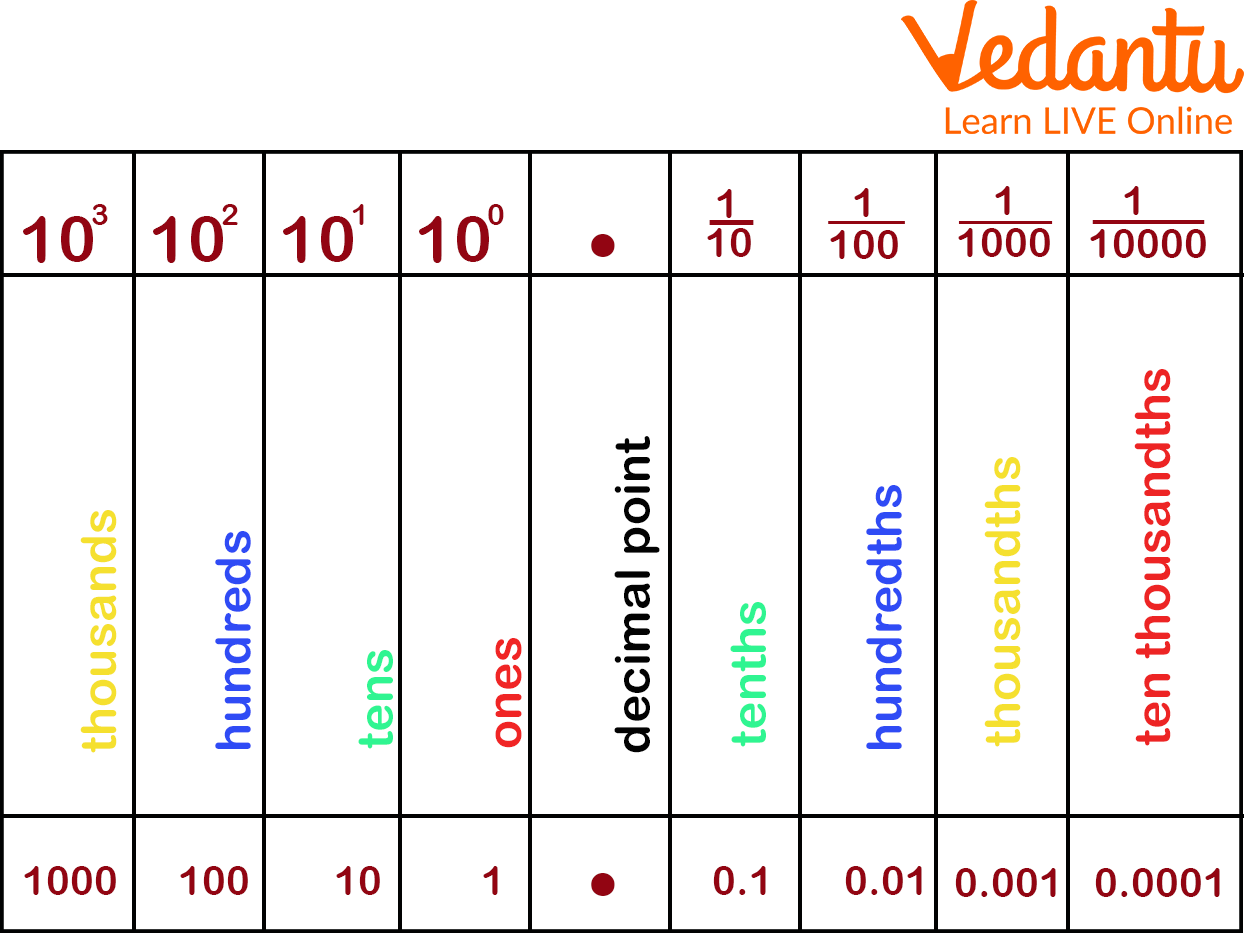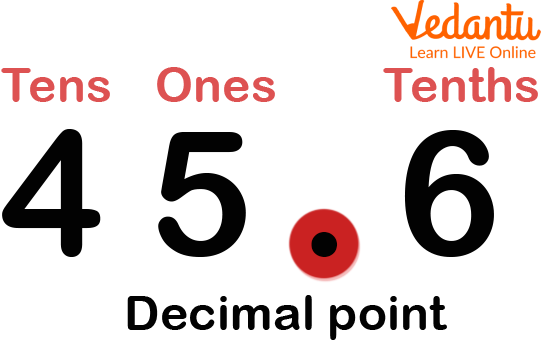Courses
Courses for Kids
Free study material
Free LIVE classes
MoreLIVE
Join Vedantu’s FREE Mastercalss

## Introduction to Addition of Decimal Number

One of the most important things we need to remember is that when you keep your math work neat, it will become very easy to add decimal numbers. So in this article, we will discuss the addition of decimals, how to add decimals with whole numbers, and many more in a very enjoyable way.## What are Decimals?

So, Decimals are the set of numbers that are written together with a dot (.) in between them and that dot is called a decimal point, which is represented in this way (.).

The decimal number is always used to express the whole number and the fraction together.

So it's very important to understand the place value of numbers while dealing with the comparison of decimals, operations on decimals, and many others which are related to decimals.Decimal Place Value Chart

We know that there is a close relationship between decimals and fractions. So, to convert any decimal number to fraction, we need to ignore the decimal point and divide the number by the place value of the last digit which is in the fractional part of the numbers.

So, In the case of decimals, which are for the whole number part, the place value system is always the same as the whole number. But after the decimal point, there opens a different world of numbers which is going on for which we use decimal fractions to represent its value.

So, When we are going towards the left, each place is ten times greater than the previous place digit. So, to the right of one's place, we have tenths $\left(\dfrac{1}{10}\right)$ and to the right of tenths, we have hundredths $\left(\dfrac{1}{100}\right)$ and it keeps on.

The addition of decimals is very similar to the regular addition of whole numbers. So, the correct way of adding decimals is to Write the numbers in the right column and to make sure that the decimal points are aligned at the correct place. And to keep the decimal point (.) in your sum you need to place a decimal point directly below decimal points in the addends.

For Example: Solve: 0.09 + 3.9 + 0.7

Ans: Now, Align the decimal point at the correct place in the sum with the decimal point in the addends, then add a zero if it's necessary. After that add the numbers from right to left.Thus, 0.09 + 3.9 + 0.7 = 4.96

Make sure that When using a calculator to add decimals, estimate the sum by rounding each of the numbers to the same place value or to its nearest whole number. So You can then easily check the calculator's result against your estimate.

Just like normal numbers, Decimal numbers are also read left to right.

So reading numbers always start with a number that's to the left of the decimal place. Which is the whole number.

So, If there is no number before the decimal point (.) just say "zero."

For example, let's say the number is 3.69, start by saying three.

If there is a zero in front of the decimal point then some people skip saying "zero" altogether and just go straight into saying "point" for the decimal point.

So to read the decimal, read the whole number part followed by "and", then read the fractional part in the same way as we read whole numbers but followed by the place value of the last digit. For example, 12.87 is "twelve and eighty-seven hundredth".## The Rule for the Addition of Decimals

While adding decimal numbers we need to remember some of the rules that would be helpful to make the addition of decimals easier.

• Align each decimal number in such a nice and clean way that they are placed in the correct place according to their place values.

• Add zeros in the places where the length of the decimal numbers is not the same.

• Always remember to convert the decimal numbers to like decimals. Wherever you needed.

• And at last, add the numbers and place the decimal point, with the given decimal points.

So, If we observe the given below decimals, we see that they are like decimals,

That's Why we will just place them in order and do the regular addition.

For exampleThen, after adding the decimal number, we get the sum as 5.98.

## Free Worksheet

Just reading things does not work as learning so to make our learning stronger and clearer we need to practice more. There are some practice sheets below in this sheet you will get questions on adding decimals, adding whole numbers, and decimals, etc.

Find the Sum:

1. 1 + 0.02 (Ans: 1.02)

2. 5 + 5.5 (Ans: 10.5)

3. 9 + 10.6 (Ans: 19.6)

4. 10 + 100.64 (Ans: 110.64)

5. 200 + 220.12 (Ans: 420.12)

## Summarykids Learning

Last updated date: 20th Sep 2023
Total views: 93.3k
Views today: 0.93k

## FAQs on Addition of Decimal Numbers

1. How to Round Decimals?

Look at the digit immediately to the right of the decimal point you wish to round up or down. Round down the digit to the left if it is less than 5, and round up the digit to the left if it is 5 or greater. For instance, 3.28 can be obtained by rounding the decimal 3.284 to the nearest hundredths.

2. Name the four operations of decimals?

Addition, subtraction, multiplication, and division. So, to add or subtract the decimal number you just line up the decimal points and after that add or subtract in the same manner as you would add or subtract the whole numbers.

3. What are the steps in adding decimal numbers?

Step 1: First of all, align the decimal numbers according to their place values.

Step 2: Convert the decimal numbers to like decimals.

Step 3: Add the numbers and place the decimal point aligned with the given decimal points.